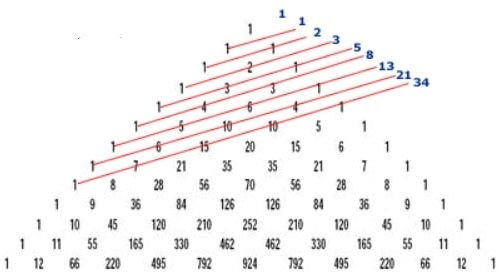# Pascal’s Triangle: GMAT Maths Secrets (Video)Pascal's Triangle represents one of the most interesting number patterns, which has intrigued mathematicians around the world for centuries.

At first Pascal’s Triangle may just look like a neatly arranged stack of numbers, but it is actually a mathematical treasure trove. Maybe you are not aware of it, but it has a lot to do with combinatorics. It also goes by different names around the world – in  India, mathematicians called it the Staircase of Mount Meru; in Iran it’s known as the Khayyam Triangle and in China as Yang Hui’s Triangle. No matter what you call it, it is certainly full of limitless patterns and secrets.

In this educational video Wajdi Mohamed Ratemi shows the infinite ways in which Pascal's Triangle works. You will be amazed by the number of mathematical combinations it can produce!

And for those of you curious to learn more on the subject, we can say that Pascal's Triangle is more than just a big triangle of numbers. There are two major areas where Pascal's Triangle is used - in algebra and in probability/combinatorics.

The triangular numbers and Fibonacci numbers can also be found in Pascal's triangle. The triangular numbers are easier to find: starting with the third number down on the left-hand edge, go down to your right and you get 1, 3, 6, 10, etc.The Fibonacci numbers are harder to locate. To find them you need to go diagonally upwards: you're looking for 1, 1, 1+1, 1+2, 1+3+1, 1+4+3, 1+5+6+1.